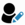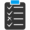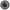Core Java - Interview Questions and Answers for '== and equals' | Search Java Interview Question - javasearch.buggybread.com# Search Interview QuestionsMore than 3000 questions in repository.There are more than 900 unanswered questions.Have a video suggestion.
Click Correct / Improve and please let us know.
Label / Company      Label / Company / TextSubmit Question

Core Java - Interview Questions and Answers for '== and equals' - 2 question(s) found - Order By NewestQ1. What is wrong with the following if statement ?

if(x==y && x.equals(y) {
}
Core Java2016-07-28 13:45:21Ans. x==y means that both references have same type and are pointing to same memory location and hence would always mean that they have same value.

x.equals(y) is not required in this case.Help us improve. Please let us know the company, where you were asked this question :LikeDiscussCorrect / Improveif statement  control statements  == and equals  ==  .equals   code optimizationTry 2 Question(s) TestRelated QuestionsDifference between == and .equals() ?What is the difference between = and == in Java ?How is == operator different for objects and primitive types ?What will be the output of following code

Integer x = 1;
Integer y = 2;
System.out.println(x == y);

What if you change 1 to "1" and Integer to String?Q2. What is wrong with the following if statement ?

if(x==y || x.equals(y) {
}
Core Java2016-07-28 13:48:23Ans. if x==y turns out to be true x.equals(y) will be true too. If x.equals(y) could be true even if x==y is true or not.

So the only possible outcomes are

1 || 1 = 1
0 || 1 = 1
0 || 0 = 0

i.e the outcome of x.equals(y)

check for x==y is not required in this if statement.Help us improve. Please let us know the company, where you were asked this question :LikeDiscussCorrect / Improveif statement  control statements  == and equals  ==  .equals   code optimizationTry 1 Question(s) TestRelated QuestionsDifference between == and .equals() ?What is the difference between = and == in Java ?How is == operator different for objects and primitive types ?What will be the output of following code

Integer x = 1;
Integer y = 2;
System.out.println(x == y);

What if you change 1 to "1" and Integer to String?

## Help us and Others Improve. Please let us know the questions asked in any of your previous interview.

Any input from you will be highly appreciated and It will unlock the application for 10 more requests.